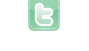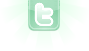## How to recognize left-clicking even if the mosue isn't moving...

For my code...
```MouseInfo m = getWorld().getMouseInfo();
if(m != null && m.getButton() == 1) {
instructions...
instructions...
instructions...
}```
I use this. The problem is though, that since I have used the if(m != null) {}, If I don't move the mouse and click, it will only do the instructions 2 times, one for clicking, and one for letting go of my finger... how do I do it so that it does the instructions infinitely even if the mouse is not being moved when clicked? Thanks...
Use this Code:
```if(Greenfoot.mouseClicked(null))
{
MouseInfo M = Greenfoot.getMouseInfo();
if(M.getButton()==1)
{
[...]
}
}```
If I understand the question right, you want to keep doing something while the mouse button is down? Just track the button state in a separate instance variable. Inside your class (outside the method!), declare an instance variable as follows:
```// Declare an instance variable:
private boolean mouseButtonDown;
```
Then change your existing code to:
```MouseInfo m = getWorld().getMouseInfo();
if(m != null) {
mouseButtonDown = (m.getButton() == 1);
}

if (mouseButtonDown) {
instructions...
instructions...
instructions...
}
```
First of all, davmac, you're a genius. Second, sorry for typing mouse wrong I was trying to type quickly. Would your code be the same as the following?
```MouseInfo m = getWorld().getMouseInfo();
if(m != null && m.getButton() == 1) {
mouseButtonDown = true;
}

if (mouseButtonDown) {
instructions...
instructions...
instructions...
} ```
Correction: There also has to be an else "mouseButtonDown = false;".
Thankyou, I don't get called a genius everyday! That's right, there also has to be an else "mouseButtonDown = false"; I think you would need to write it something like this:
```MouseInfo m = getWorld().getMouseInfo();
if(m != null) {
if (m.getButton() == 1) {
mouseButtonDown = true;
}
else {
mouseButtonDown = false;
}
}

if (mouseButtonDown) {
instructions...
instructions...
instructions...
}
```
I have one more problem... I am making this game where a rocket starts in the middle of a grid, and it's location is 0, 0. There are three different planets in different locations, such as -1, 0 & 5, 2 & etc. What I would like to do is set a random location for all 3 planets. I have done so like this. For the following code, p stands for planet.
```p1x = Greenfoot.getRandomNumber(21) - 9;
if(p1x < 1) {
p1x--;
}
p1y = Greenfoot.getRandomNumber(21) - 9;
if(p1y < 1) {
p1y--;
}
p2x = Greenfoot.getRandomNumber(21) - 9;
if(p2x < 1) {
p2x--;
}```
& so on.... Instead of this... I want to set up a public void randomInt(String varname) {} So all I have to do is put the name of my variable in the method, and a random number between -10 & 10, not counting 0, is entered into the variable. The problem is, how do I de-string the String varname so that I can do the follozing?:
```varname = Greenfoot.getRandomNumber(20) - 9;
if(varname < 1) {
varname--;
}```
You can't do that exactly in Java. You have a few options: First option: store the values in a HashMap eg:
```public void randomInt(HashMap<String,Integer> varMap, String varname)
{
int var = Greenfoot.getRandomNumber(20) - 9;
if (var < 1) {
var--;
}
varMap.put(varname, var);
} ```
For this to work you'll need to import the HashMap class at the top of your class:
```import java.util.HashMap;
```
You'll also have to create a new map (new HashMap<String,Integer>()) and pass it to the randomInt method. Replace your code above with:
```HashMap<String,Integer> varMap = new HashMap<String,Integer>();
randomInt(varMap, "p1x");
randomInt(varMap, "p1y";
randomInt(varMap, "p2x";
...
```
To get values out of the map, use "hashmap.get(varname)" (where "varname" is a String). Second option: use an array:
```int [] planetCoords = new int;  // 3 x and 3 y coordinates
for (int i = 0; i < 6; i++) {
randomInt(planetCoords, i);
}
```
and
```public void randomInt(int [] array, int index)
{
int var = Greenfoot.getRandomNumber(20) - 9;
if (var < 1) {
var--;
}
array[index] = var;
}
```
Now the p1x value is planetCoords, p1y is planetCoords, p2x is planetCoords, etc. There are other possibilities, but this should get you started!